# 表层沉积物对水中F-吸附及解析过程的影响Analytical Experiment and Mechanism Study of Surface Sediment on Fluorine Solution

• 全文下载: PDF(1280KB)    PP.669-676   DOI: 10.12677/AEP.2019.95090
• 下载量: 283  浏览量: 401

After the surface water is polluted, it reacts with the surface sediments and releases the pollutants to form the secondary pollution source. In order to study the adsorption and desorption of F in soils and determine the chemical reactions that happened during the F analysis, kinetic tests and ad-sorption and desorption experiments were used in this study. Combined with pseudo-second-order kinetic equation and isothermal adsorption equation, the reaction kinetics and adsorption analysis between the mud and the reservoir water show that when the reaction time and the initial concen-tration are changed; the reaction rate, the reaction process and the migration and transformation rules of F in water are obtained. The results show that: 1) The kinetic process controlled by chemi-cal reaction may be the dominant surface sediment, which can be described by pseudo second-order kinetic equation. The fitting equation is y = 0.7401x + 0.1266 and the correlation coefficient is R2 = 0.9985. 2) The isotherm of F adsorption by surface sediment is in accordance with the linear relationship, and the saturated analytical volume of F is 30.56 mg•g−1. 3) After the soil sample is in contact with the fluorine solution, the pH value of the solution increases with the increase of the F-mass concentration, indicating that OH− is continuously released in the F analysis progresses; it can be seen from the change of other ions. The chemical reactions of F occurring during the analysis process are precipitation-dissolution, adsorption-analysis, and ion exchange.

1. 引言

2. 材料与方法

2.1. 试验材料Table 1. Particle analysis results and soil classificationTable 2. Physical characteristics of the test sampleTable 3. Chemical characteristics of the test soil samples

2.2. 试验方法

3. 结果与分析

${Q}_{e}=\frac{\left({C}_{e}-{C}_{0}\right)V}{m}$ (1)

3.1. 动力学试验结果分析Table 4. F− five kinds of adsorption kinetic model fitting

3.2. F解析情况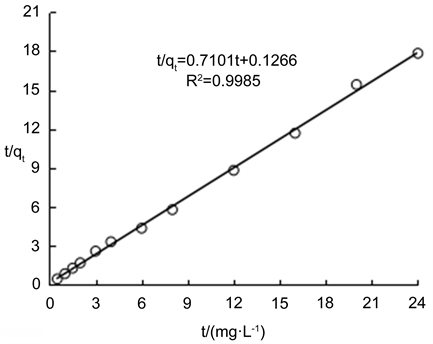Figure 1. Pseudo-second-order kinetic fit

3.3. 解析机理分析

3.3.1. 环境(pH)变化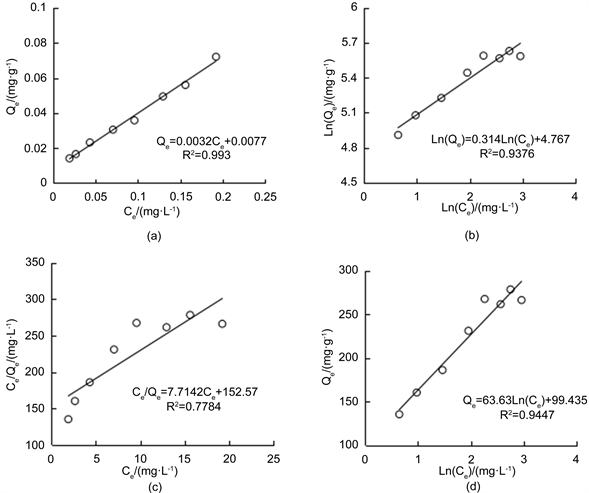Figure 2. The fitting curve of F desorption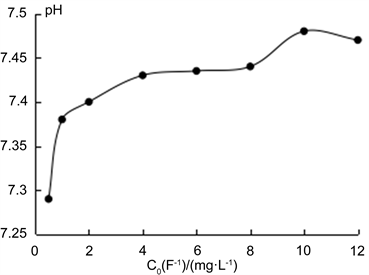Figure 3. The change of pH value with F concentration

3.3.2. 其他离子的变化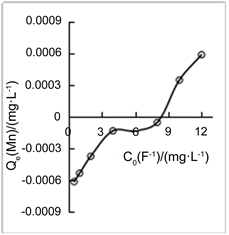(a) Fe浓度变化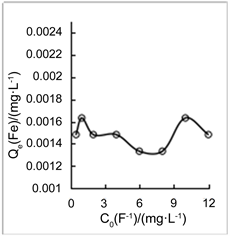(b) Mn浓度变化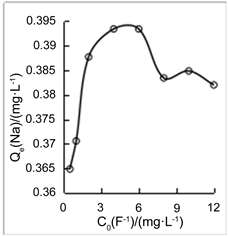(c) Na+浓度变化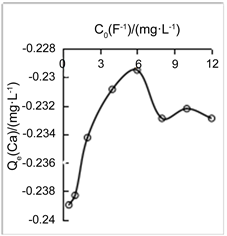(d) Ca2+浓度变化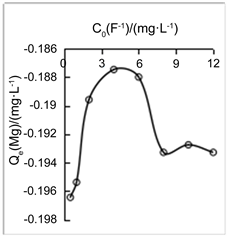(e) Mg2+浓度变化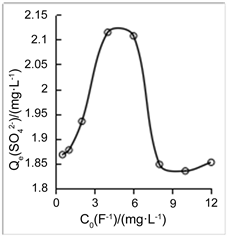(f) SO42- 浓度变化

Figure 4. Other ions with the F concentration trend

3.3.3. 吸附机理分析

4. 结论

NOTES

*第一作者。

#通讯作者。

  曹春, 康宏亮, 李萍萍, 等. 聚丙烯偕胺肟改性羊毛对氟离子的吸附及机理[J]. 高分子学报, 2016(4): 486-493.  荆秀艳, 袁周燕, 杨红斌, 等. 土氟静态吸附特性及其影响因素[J]. 生态环境学报, 2008, 17(5): 1818-1821.  杨军耀. 水–土系统氟迁移影响因素分析[J]. 工程勘察, 1998(3): 42-44.  江霜英, 高廷耀. 粘土对水中氟离子吸附去除机理的研究[J]. 化工环保, 2003, 23(4): 204-208.  王庆文. 土壤对矿井水中F−的吸附动力学和吸附机理初探[J]. 河南理工大学学报(自然科学版), 2009, 28(6): 807-810.  梁鹏. 稀土改性壳聚糖树脂的制备、表征及对氟离子的吸附特性研究[D]: [博士学位论文]. 青岛: 中国海洋大学, 2013.  阮建云, 马立锋, 石元值, 等. 茶园土壤对氟的吸附与解吸特性[J]. 茶叶科学, 2001, 21(2): 161-165.  Peek, D.C. and Volk, V.V. (1985) Fluoride Sorption and Desorption in Soils. Soil Science Society of America Journal, 49, 583-586. https://doi.org/10.2136/sssaj1985.03615995004900030010x  Zhu, M.X., Ding, K.Y., Jiang, X. and Wang, H.-H. (2007) Investigation on Co-Sorption and Desorption of Fluoride and Phosphate in a Red Soil of China. Water Air & Soil Pol-lution, 183, 455-465. https://doi.org/10.1007/s11270-007-9394-0  陈男. 天然及合成多孔性粘土材料对地下水中氟化物的吸附性能研究[D]: [博士学位论文]. 北京: 中国地质大学, 2012.  韩德刚, 高盘良. 化学动力学基础[M]. 北京: 北京大学出版社, 1987.  董岁明. 氟在土–水系统中的迁移机理与含氟水的处理研究[D]: [博士学位论文]. 西安: 长安大学, 2004.  梁秀娟. 吉林西部洋沙泡水库水土环境中氟的迁移转化机理研究[D]: [博士学位论文]. 长春: 吉林大学, 2008.  Nur, T., Loganathan, P., Nguyen, T.C., et al. (2014) Batch and Column Adsorption and Desorption of Fluoride Using Hydrous Ferric Oxide: Solution Chemistry and Modeling. Chemical Engineering Journal, 247, 93-102. https://doi.org/10.1016/j.cej.2014.03.009  王洪涛. 多孔介质污染物迁移动力学[M]. 北京: 高等教育出版社, 2008.